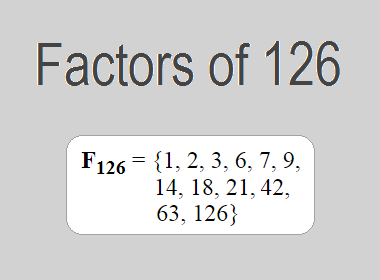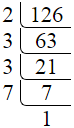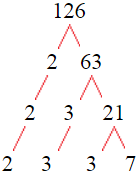# Factors of 126The factors of 126 are 1, 2, 3, 6, 7, 9, 14, 18, 21, 42, 63, and 126 i.e. F126 = {1, 2, 3, 6, 7, 9, 14, 18, 21, 42, 63, 126}. The factors of 126 are all the numbers that can divide 126 without leaving a remainder.

We can check if these numbers are factors of 126 by dividing 126 by each of them. If the result is a whole number, then the number is a factor of 126. Let's do this for each of the numbers listed above:

·        1 is a factor of 126 because 126 divided by 1 is 126.

·        2 is a factor of 126 because 126 divided by 2 is 63.

·        3 is a factor of 126 because 126 divided by 3 is 42.

·        6 is a factor of 126 because 126 divided by 6 is 21.

·        7 is a factor of 126 because 126 divided by 7 is 18.

·        9 is a factor of 126 because 126 divided by 9 is 14.

·        14 is a factor of 126 because 126 divided by 14 is 9.

·        18 is a factor of 126 because 126 divided by 18 is 7.

·        21 is a factor of 126 because 126 divided by 21 is 6.

·        42 is a factor of 126 because 126 divided by 42 is 3.

·        63 is a factor of 126 because 126 divided by 63 is 2.

·        126 is a factor of 126 because 126 divided by 126 is 1.

## How to Find Factors of 126?

1 and the number itself are the factors of every number. So, 1 and 126 are two factors of 126. To find the other factors of 126, we can start by dividing 126 by the numbers between 1 and 126. If we divide 126 by 2, we get a remainder of 0. Therefore, 2 is a factor of 126. If we divide 126 by 3, we get a remainder of 0. Therefore, 3 is also a factor of 126.

Next, we can check if 4 is a factor of 126. If we divide 126 by 4, we get a remainder of 2. Therefore, 4 is not a factor of 126. We can continue this process for all the possible factors of 126.

Through this process, we can find that the factors of 126 are 1, 2, 3, 6, 7, 9, 14, 18, 21, 42, 63, and 126. These are the only numbers that can divide 126 without leaving a remainder.

********************

********************

## Properties of the Factors of 126

The factors of 126 have some interesting properties. One of the properties is that the sum of the factors of 126 is equal to 312. We can see this by adding all the factors of 126 together:

1 + 2 + 3 + 6 + 7 + 9 + 14 + 18 + 21 + 42 + 63 + 126 = 312

Another property of the factors of 126 is that the prime factors of 126 are 2, 3, and 7 only.

## Applications of the Factors of 126

The factors of 126 have several applications in mathematics. One of the applications is in finding the highest common factor (HCF) of two or more numbers. The HCF is the largest factor that two or more numbers have in common. For example, to find the HCF of 126 and 84, we need to find the factors of both numbers and identify the largest factor they have in common. The factors of 126 are 1, 2, 3, 6, 7, 9, 14, 18, 21, 42, 63, and 126. The factors of 84 are 1, 2, 3, 4, 6, 7, 12, 14, 21, 28, 42, and 84. The largest factor that they have in common is 42. Therefore, the HCF of 126 and 84 is 42.

Another application of the factors of 126 is in prime factorization. Prime factorization is the process of expressing a number as the product of its prime factors. The prime factors of 126 are 2, 3, and 7, since these are the only prime numbers that can divide 126 without leaving a remainder. Therefore, we can express 126 as:

126 = 2 × 3 × 3 × 7

We can do prime factorization by division and factor tree method also. Here is the prime factorization of 126 by division method,126 = 2 × 3 × 3 × 7

Here is the prime factorization of 126 by the factor tree method,126 = 2 × 3 × 3 × 7

## Conclusion

The factors of 126 are the numbers that can divide 126 without leaving a remainder. The factors of 126 are 1, 2, 3, 6, 7, 9, 14, 18, 21, 42, 63, and 126. The factors of 126 have some interesting properties, such as having a sum of 312. The factors of 126 have several applications in mathematics, such as finding the highest common factor and prime factorization.